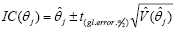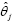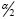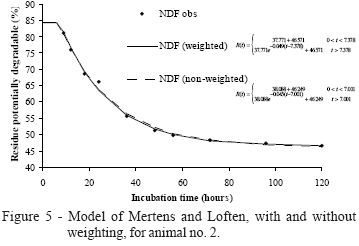# Abstracts

Degradation models exhibit a non-linear behavior and the selection of a model to describe the degradability depends on the coherence of the model with the involved biological events. The purpose of this study is to evaluate the behavior of the parameters of the degradation model proposed by Mertens & Loften, adjusted to the results of an in situ degradability trial. The experiment evaluated the potential degradable residue of neutral detergent fiber (NDF) of coastcross grass (Cynodon dactylon × Cynodon nlemfuensis) submitted to two cutting ages (30 and 90 days), with three replicates. For each cutting age, the potentially degradable residue of NDF was studied using fifteen incubation times (0; 0,5; 1; 3; 6; 9; 12; 18; 34; 35; 48; 56; 72; 96 e 120 hours). The experimental unit comprised one non-lactating cow with a permanent ruminal fistula. Mean and individual adjustments were obtained for the animals in three different configurations: inverse variance weight without autoregressive errors; unweighted with autoregressive errors, and unweighted without autoregressive errors. Variances of parameter estimators were also obtained by means of the mean parameter covariance matrix, providing expressions for the estimation of the confidence age for the parameters of the model. A weighting of the model by the inverse variance resulted in estimates statistically equal to zero for the colonization time. The use of a structure of second order autoregressive errors improved the fit of the model of Mertens & Loften, providing more precise estimates of the parameters.

ruminal degradability; non-linear regression; parameters estimation; coast-cross grass

STATISTICS

A study of in situ degradability: heterogeneity of variances and correlated errors

Taciana Villela SavianI; Joel Augusto MunizII,* * Corresponding author < joamuniz@ufla.br>

IUFLA - Programa de Pós-Graduação em Agronomia/Estatística e Experimentação Agropecuária

IIUFLA - Depto. de Ciências Exatas, C.P. 3037 - 37200-000, Lavras, MG - Brasil

ABSTRACT

Degradation models exhibit a non-linear behavior and the selection of a model to describe the degradability depends on the coherence of the model with the involved biological events. The purpose of this study is to evaluate the behavior of the parameters of the degradation model proposed by Mertens & Loften, adjusted to the results of an in situ degradability trial. The experiment evaluated the potential degradable residue of neutral detergent fiber (NDF) of coastcross grass (Cynodon dactylon × Cynodon nlemfuensis) submitted to two cutting ages (30 and 90 days), with three replicates. For each cutting age, the potentially degradable residue of NDF was studied using fifteen incubation times (0; 0,5; 1; 3; 6; 9; 12; 18; 34; 35; 48; 56; 72; 96 e 120 hours). The experimental unit comprised one non-lactating cow with a permanent ruminal fistula. Mean and individual adjustments were obtained for the animals in three different configurations: inverse variance weight without autoregressive errors; unweighted with autoregressive errors, and unweighted without autoregressive errors. Variances of parameter estimators were also obtained by means of the mean parameter covariance matrix, providing expressions for the estimation of the confidence age for the parameters of the model. A weighting of the model by the inverse variance resulted in estimates statistically equal to zero for the colonization time. The use of a structure of second order autoregressive errors improved the fit of the model of Mertens & Loften, providing more precise estimates of the parameters.

Key words: ruminal degradability, non-linear regression, parameters estimation, coast-cross grass

RESUMO

INTRODUCTION

Waldo, in 1970, was the first to show that degradation profiles were combinations of digestible and indigestible materials and that the potentially digestible fraction follows a first order kinetics (Mertens, 1993). The neutral detergent fiber (NDF) digestion exhibits a period in which effectively a degradation of the component does not occur (Mertens, 1977). Mertens & Loften (1980) have suggested theinclusion of a parameter for the parameter estimates of the first order model of Waldo et al. (1972) which examines this period, for the degradability in situ and in vitro of the NDF.

Several important statistical considerations, normally not taken into account in the study of ruminal degradation curves, are the heterogeneity the variance and the existence of autocorrelation between the adjustment residues. If such aspects are ignored in the adjustment process, respective to these facts, biased estimates and an underestimation of the variances of the parameters may result (Souza, 1998). In accordance with Hoffman & Viera (1998), in the presence of the heterogeneity of variance, the use of the weighted least squares method is more adequate for forming estimates that are unbiased and of minimum variance; in addition, in the presence of variance heterogeneity and autocorrelation of the residues, the generalized least squares method is more efficient than the weighted and ordinary least squares method.

In the case of the study of nonlinear models that describe ruminal degradation, it is reasonable to incorporate autocorrelation, seeing that the means of degradation of the actual nutritional component of interest are taken from one animal, and, therefore, probably correlated.

This research evaluates the behavior of the parameter estimate for the ruminal degradability model proposed by Mertens & Loften (1980) and obtains expressions for the variance of its parameter estimators, which takes into consideration an autoregressive error structure weighted for the inverse of variance, in this manner, tests for the actual existence of biologically interpretable parameters in the model.

MATERIAL AND METHODS

To illustrate the methodology, this study makes use of experimental data (Reis, 2000) related to the potentially degradable residue of neutral detergent fiber (NDF) of coastcross grain (Cynodon dactylon ×Cynodon nlemfuensis) subjected to two different cutting ages (30 and 90 days). For each cutting age, the degradation profile was evaluated for fifteen incubation times (0; 0.5; 1; 3; 6; 9; 12; 18; 24; 36; 48; 56; 72; 96 and 120 hours), the experimental unit consisting of a non-lactant cow with a permanent ruminant fistula.

The model used to describe the potentially degradable residue was that of Mertens & Loften (1980), as shown by:,

in which : R(t) is the residue after incubation in the rumen at time t (%); D is the degradable fraction (%); c is the degradation rate (hours-1); t is the incubation time (hours); I is the insoluble and non-degradable fraction (%) and L is the colonization time or lag time (hours).

Parameter estimates for the individual curves and parameter estimates for the mean curve were obtained by means of: ordinary least squares (OLS), when the residual structure does not violate any of the presuppositions; weighted least squares (WLS), when the assumption of homogeneity of variances was violated and generalized least squares (GLS) when the assumption of residual independence was violated. In the process of estimation of the parameters of the nonlinear model, the solution for the systems of nonlinear normal equations was obtained by the iterative Gauss-Newton method.

In the case of weighted nonlinear models, the "Weight" option of the proc model was employed, and, in order to verify the presence of residual autocorrelation, the macro %AR(y,p) was used, implemented in the same module (SAS, 1995), together with the analysis of correlograms. Only the animals that displayed autocorrelation of the first or second order and when the weighting was necessary, were used in the adjustments.

The estimates of the asymptotic covariance matrix are presented by Draper & Smith (1998) in the following form:, in which; X is the matrix of the partial derivatives of the model in relation to the parameters, and QME is the residual mean square. In this form the confidence age was defined by the parameter qj as:in which:is the estimate of the j-th parameter of the model;is the highest percentileof the Student t-distribution for the degree of freedom of the residue andis the estimate of the variance of the estimate of the j-th parameter.

RESULTS AND DISCUSSION

The increase in incubation time of the samples in the rumen is related to a decrease in the residual variances of the degradation of NDF of the coast-cross grass (Table 1). Vieira (1995) has shown that the model of Mertens & Loften (1980), linearized by logarithmic transformation, tends to present mean deviations that approached zero for the first incubation time, with the precision diminishing over greater times. A similar behavior was observed for grass cut at age of 90 days.

The relation between the greatest and the least variance was 2.54 for grass harvested at 30 days and does not display heterogeneity. For the grass cut at 90 days, the least variance is 9.13 times less than the greatest variance, a relationship that is significant for three experimental groups (three animals) and 14 degrees of freedom, with a significance level of 5%, according to Hartley's maximum F-ratio (Pearson & Hartley, 1970).

Studying growth curves of bovines, Mazzini et al. (2005) and Silva et al. (2002), indicate that weighting by inverse variance is a viable solution for the occurrence of variance heterogeneity, yet it does not solve the problem of residual autocorrelation, which requires the use of procedures with an autoregressive error structure. The ratio between the maximum and minimum variance was used by Mazzini (2001) to study growth curves of Hereford cattle. He indicates, to the extent that the age of the animals increased, there was an increment in the variances of the body weight, this being also another pattern of heteroscedascity.

The autocorrelation parameters (f1 e f2) are only significant when using a significance level of 5%, for animal number 2, for the 30 day cutting age, adjusting, therefore, a structure of second order autoregressive errors AR(2) (Table 2).

When examining residual autocorrelation, Mazzini (2001), Mazzini et al. (2003; 2005) studied the growth curves of bovines by means of various functions and found that, in relation to the fitting of the mean curves, some functions did not fit an autoregressive model of first or second order; however, when fitting individual curves, there were animals that displayed these error structures.

By means of a partial autocorrelation function for the Mertens & Loften (1980) model without weighting and without AR, it can be seen that the residues follow an autoregressive error structure AR2 (Figure 1). For this same configuration, adjusting the same function and considering the error structure AR2, it may be seen, by the function of the autocorrelation, that the residues become independent (Figure 2).

The parameters of the degradation model proposed by Mertens & Loften (1980) (Table 3) are estimated without taking into consideration the weight and structure of the correlated errors, for each animal as well as for the mean. For the adjustment of the mean curve, an increase was found in the value of the estimate of the colonization time (parameter L) for a grass cutting age of 90 days, probably because in plants of an advanced age there is a loss of water and a strong lignin complex with the components of the cellular wall. For the digestion process to occur, the microorganisms must penetrate the resistant barriers of the surface of the food particles to reach their preferred substrata, and the degree to which the microorganisms fix themselves and penetrate these physical barriers, reflects on the colonization time.

With regard to the values of the parameter estimates, Feitosa (1999), in comparing models in tests of degradability in situ with coast-cross grass hay, observed that the model of McDonald (1981), also corrected for the colonization time, resulted in the same estimates as the model of Mertens & Loften (1980). McDonald (1981) gives values of 43.26% for the insoluble and nondegradable fraction; 4%.h-1 for the rate of degradation (parameter c) and 2.45 hours for the colonization time. This model was also used by Lira (2000) to predict the degradation of NDF of brachiaria grass (Brachiaria decumbens Stapf.) in two seasons (dry and rainy), showing mean values of 51.32% for the degradable fraction; 38.08% for the insoluble and nondegradable fraction; 2.5% h-1 for the rate of degradation and 7.64 hours for the colonization time in the rainy season.

According to the significant values for the autocorrelation estimates of the parameters (Table 2), the model was adjusted only for animal 2, for the grass cutting age of 30 days, with a second order autoregressive error structure AR(2). In Figure 3 it is possible to observe the data adjustment to the model of Mertens & Loften (1980), whether or not the second order autoregressive error structure was used.

Values of the estimates of the parameters, in considering the error structure AR(2), do not undergo large changes (Table 4). The estimates of the variance of the parameter estimate, obtained by the variance-covariance matrix for this same configuration, shows significant reduction in their values, resulting in confidence ages of less amplitude and more precise parameter estimates. This methodology was also used by Pereira et al. (2005) for the prediction of mineralized nitrogen in latosols by means of nonlinear models. In comparing confidence ages obtained by means of a variance-covariance matrix of parameters, the author also established lower estimates of parameter variance.

With the increase in incubation time of the samples in the rumen, there was a decrease in the residual variances of NDF of the potential degradable residues of the coastcross grass for the cutting age of 90 days (Table 1). The parameter estimates for the Mertens & Loften (1980) model are in Table 5, with and without weighting, with the respective variance estimates obtained for the parameter covariance matrix for each animal and their mean. The adjustment of Mertens & Loften (1980) model to the data, whether or not weighting is used, can be seen in Figures 4 and 7 , for each one of the animals and their mean, respectively.In the curve adjustment for animals 1 and 3 (Table 5), the values of parameter estimates referring to the non-degradable fraction (parameter I), rate of degradation (parameter c) and colonization time (parameter L) undergo reductions when weighting is introduced into the model. For this last parameter, the reduction together with the increase of the variance estimate rise to nonsignificant, signifying that the potentially degradable residue of NDF of coastcross grass begins to undergo substantial losses as soon as incubated in the rumen of these animals, that is, for an incubation time equal to zero.

For the adjustment of the curve for animal 2 (Table 5), the inverse behavior was observed by means of weighting, where the values of the parameters estimates referring to the non-degradable fraction (parameter I), rate of degradation (parameter c) and the colonization time (parameter L) underwent increases, while their variance estimates were lower, resulting in confidence ages of less amplitude.

For the adjustment of the mean curve of the animals, the values indicate that, only for the non-degradable fraction (parameter I), the weighting resulted in a lower variance estimate and, consequently, in a confidence age of lower amplitude. For the other parameters of the model, when using weighting, there was an increase in the variance estimates and ages with greater amplitude.

CONCLUSIONS

The weighting of the model, for the inverse ofthe variance, provides less precise estimates for thecolonization time, when the coastcross grass was processed in advanced ages. The use of a second order autoregressive error structure improved the adjust ments to the model of Mertens & Loften (1980), providing more precise estimates for the parameters.

Accepted April 13, 2007

• DRAPER, N.R.; SMITH, H. Applied regression analysis. 3.ed. New York: J. Wiley & Sons, 1998. 706p.
• FEITOSA, J.V. Ensaios de degradabilidade in situ: uma abordagem estatística. Jaboticabal: Unesp, 1999.117p. (Dissertação - Mestrado).
• HOFFMANN, R.; VIEIRA, S. Análise de regressão: uma introdução à econometria. 3.ed. São Paulo: HUCITEC, 1998. 379p.
• LIRA, V.M.C. Utilização de diferentes modelos matemáticos e marcadores para simulação da cinética digestiva e de trânsito do capim braquiária (Brachiaria decumbens Stapf.). Viçosa: UFV, 2000. 90p. (Dissertação - Mestrado).
• MAZZINI, A.R. de A. Análise da curva de crescimento de machos Hereford considerando heterogeneidade de variâncias e autocorrelação dos erros. Lavras: UFLA, 2001. 94p. (Dissertação - Mestrado).
• MAZZINI, A.R. de A.; MUNIZ, J.A.; AQUINO, L.H. de; SILVA, F.F. e. Análise da curva de crescimento de machos Hereford. Ciência e Agrotecnologia, v.27, p.1105-1112, 2003.
• MAZZINI, A.R. de A.; MUNIZ, J.A.; SILVA, F.F. e; AQUINO, L.H. de Curva de crescimento de novilhos Hereford: heterocedasticidade e resíduos autoregressivos. Ciência Rural, v.35, p.422-427, 2005.
• McDONALD, I. A revised model for the estimation of protein degradability in the rumen. Journal of Agricultural Science, v.96, p.251-252, 1981.
• MERTENS, D.R. Dietary fiber components: relatioship to the rate and extent of ruminal digestion. Federation Proceedings, v.36, p.187-192, 1977.
• MERTENS, D.R. Rate and extent of digestion. In: FORBES, J.M.; FRANCE, J. (Ed.). Qualitative aspects of ruminant digestion and metabolism Wallingford: Cambridge University, 1993. cap.2, p.13-51.
• MERTENS, D.R.; LOFTEN, J.R. the effects of starch on forage fiber digestion kinetics in vitro Journal of Dairy Science, v.63, p.1437-1446, 1980.
• PEARSON, E.S.; HARTLEY, H.O. Biometrika tables for statisticians. 3.ed. Cambridge: Cambridge University, 1970.
• PEREIRA, J.M.; MUNIZ, J.A.; SILVA, C.A. Non linear models to predict nitrogen mineralization in na oxisol. Scientia Agricola, v.62, p.395-400, 2005.
• REIS, S.T. dos. Valor nutricional de gramíneas tropicais em diferentes idades de corte. Lavras: UFLA, 2000. 99p. (Dissertação - Mestrado).
• SAS INSTITUTE Inc. SAS/ETS® User's guide. Version 6. 2.ed. Cary, 1995.
• SILVA, F. F. e; AQUINO, L.H. de; OLIVEIRA, A.I.G. de Estimativas de parâmetros genéticos em curvas de crescimento de gado nelore. Ciência e Agrotecnologia, v.26, p.1562-1567, 2002.
• SOUZA, G. da S. Introdução aos modelos de regressão linear e não-linear. Brasília: EMBRAPA-SPI; EMBRAPA-SEA, 1998. 489p.
• VIEIRA, R.A.M. Modelos matemáticos para estimativa de parâmetros da cinética de degradação do capim elefante (Pennisetum purpureum, Schum. cv. Mineiro) em diferentes idades de corte. Viçosa: UFV, 1995. 88p. (Dissertação - Mestrado).
• WALDO, D.R.; SMITH, L.W.; COX, L.E. Model of cellulose disappearance from the rumen. Journal of Dairy Science, v.55, p.125-129, 1972.
•• *
Corresponding author <

# Publication Dates

• Publication in this collection
05 Oct 2007
• Date of issue
Oct 2007